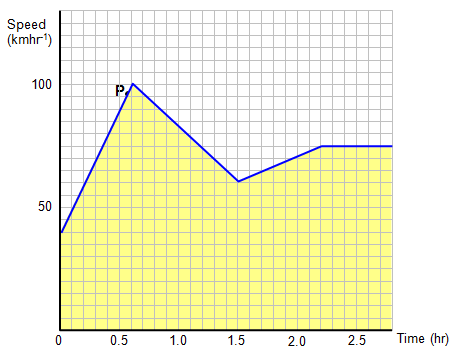## Instantaneous and average speed, velocity and acceleration

When we think about the speed of an object it is important to understand that there are various ways of expressing this.

(a) Average value of a quantity
Imagine that you go on a journey of 188.25 km and that this takes 2.8 hours.

If we consider the whole journey and used the formula speed = total distance/total time we would get a speed of 188.25/2.8 = 67.23 kmhr-1. We call this value your average speed for the journey. It does not mean that you travelled at this speed for the whole journey or even for very much of it. (N.B multiplying your average speed by the total tim will give you the total distance travelled =188.25 km).

(b) Instantaneous value of the quantity
However, if at a certain point on the journey you look at the speedometer and see that you are travelling at 96 kmhr-1 (60 mph). We call this value your instantaneous speed as it is your speed at that particular instant (marked P on the graph). It would be found by taking the short time (Dt) it takes the car to travel a short distance (Ds). As before it does not mean that you travelled at this speed for the whole journey or even for very much of it – it is simply your speed at that particular moment.

Both these quantities can be considered using the following speed-time graph.Total time = 2.8 hours
Total distance (area under the speed- time curve)= 188.25 km
Average speed = total distance/total time = 67.23 kmhr- 1

A similar argument can be used to consider average and instantaneous values of velocities and accelerations.

A VERSION IN WORD IS AVAILABLE ON THE SCHOOLPHYSICS USB

© Keith Gibbs 2020